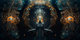cancel
Showing results for
Did you mean:

Fabric is Generally Available. Browse Fabric Presentations. Work towards your Fabric certification with the Cloud Skills Challenge.New Member

## Dax TIME function bug with Hour = 13

with hour = 14, all is OK :

``````define
table myMinute = {12}
Evaluate
{ ("Hour", 14),
("myMinute", SELECTEDVALUE(myMinute[Value])),
("check in TIME", TIME(14, SELECTEDVALUE(myMinute[Value]), 0) = TIME(14,12,0))
}``````

=>with hour = 13, it is KO

``````define
table myMinute = {12}
Evaluate
{ ("Hour", 13),
("myMinute", SELECTEDVALUE(myMinute[Value])),
("check in TIME", TIME(13, SELECTEDVALUE(myMinute[Value]), 0) = TIME(13,12,0))
}``````

=>1 ACCEPTED SOLUTIONSuper User

Hi @cturle

This is indeed confusing since, on the face of it, the two time values should be equal 😞

Since Date/Time values are stored as Decimal values , the values are stored approximately, and any equality-related comparisons can return unexpected results.

https://learn.microsoft.com/en-us/power-bi/connect-data/desktop-data-types#datetime-types

https://learn.microsoft.com/en-us/power-bi/connect-data/desktop-data-types#accuracy-of-number-type-c...

In your second example, you can verify that there is a difference in the Decimal values stored by subtracting the two values and formatting in scientific notation for example, giving a difference of approximately -1.1102E-16.

I can't explain in this specific case why the two time values are stored as slightly different values, other than point to the above documentation. Using SELECTEDVALUE to return a value from a (query) table presumably plays a role. Perhaps someone out there can explain the specifics in this case! 🙂

Because of all this, for any equality-related comparisons of Date/Time or Decimal values, I would suggest either rounding the values or testing whether ABS ( difference ) < threshold.

e.g.

``````DEFINE
TABLE myMinute = {
12
}
VAR MyHour = 13
VAR Threshold = 1E-6 -- small threshold
VAR RoundingMultiple = 1E-6 -- small multiple for rounding

EVALUATE
VAR Time1 =
TIME ( MyHour, SELECTEDVALUE ( myMinute[Value] ), 0 )
VAR Time2 =
TIME ( MyHour, 12, 0 )
RETURN
{
( "Hour", MyHour ),
( "myMinute", SELECTEDVALUE ( myMinute[Value] ) ),
( "check in TIME", Time1 = Time2 ),
( "check in TIME threshold", ABS ( Time1 - Time2 ) < Threshold ),
( "check in TIME rounded", MROUND ( Time1, RoundingMultiple ) = MROUND ( Time2, RoundingMultiple ) )
}``````

Regards

Owen Auger
Blog
3 REPLIES 3New Member

Can you run

``````Measure1 = TIME(13, SELECTEDVALUE(myMinute[Value]), 0)
Measure2 = TIME(13, 12, 0)
MeasureCheck = IF(Measure1 = Measure2, "OK", "KO")``````

If Measure1 and Measure2 are not equal, it suggests that the issue likely resides in how the TIME function is processing the hour value of 13 or how it's interacting with SELECTEDVALUE(myMinute[Value]).

If they are equal but your original expression still fails, the issue might be related to how the equality check is being performed in your original DAX expression.New Member

Hi Owen,

As you said, Time values are stored approximately as floating point numbers. This is why in my example, i don't store any Time value. I have no Time1 nor Time2. I only store an integer value (12). So "a priori" this is not the root problem, but ...

I have simplified again the problem removing the call to SelectedValue :

``````define
var T1 = TIME(13, max(rand(),12), 0)
var T2 = TIME(13,12,0)
var T3 = TIME(13, max(1,12), 0)
Evaluate
{ ("", 0),
("case minutes=max(rand(),12) : T1 - 0.55", T1 - 0.55),
("case minutes=12             : T2 - 0.55", T2 - 0.55),
("case minutes=max(1,12)      : T3 - 0.55", T3 - 0.55)
}``````Now, it is clearer. What i understand is that there exists a "pre-processor" which computes T2 ans T3 at "compile time" to optimize the code. These results are surely stored somewhere and, as you said, precision is lost. T1 is computed at "runtime" and is not stored ! => this is why T1 - 0.55 <> 0

So the diff seems to come from a test between a (stored, pre-processed) approximate value and a (not stored, computed) precise value

=> Loss of precision due to storing a decimal value is the technical root cause. You're right 🙂

Thanks and have a good daySuper User

Hi @cturle

This is indeed confusing since, on the face of it, the two time values should be equal 😞

Since Date/Time values are stored as Decimal values , the values are stored approximately, and any equality-related comparisons can return unexpected results.

https://learn.microsoft.com/en-us/power-bi/connect-data/desktop-data-types#datetime-types

https://learn.microsoft.com/en-us/power-bi/connect-data/desktop-data-types#accuracy-of-number-type-c...

In your second example, you can verify that there is a difference in the Decimal values stored by subtracting the two values and formatting in scientific notation for example, giving a difference of approximately -1.1102E-16.

I can't explain in this specific case why the two time values are stored as slightly different values, other than point to the above documentation. Using SELECTEDVALUE to return a value from a (query) table presumably plays a role. Perhaps someone out there can explain the specifics in this case! 🙂

Because of all this, for any equality-related comparisons of Date/Time or Decimal values, I would suggest either rounding the values or testing whether ABS ( difference ) < threshold.

e.g.

``````DEFINE
TABLE myMinute = {
12
}
VAR MyHour = 13
VAR Threshold = 1E-6 -- small threshold
VAR RoundingMultiple = 1E-6 -- small multiple for rounding

EVALUATE
VAR Time1 =
TIME ( MyHour, SELECTEDVALUE ( myMinute[Value] ), 0 )
VAR Time2 =
TIME ( MyHour, 12, 0 )
RETURN
{
( "Hour", MyHour ),
( "myMinute", SELECTEDVALUE ( myMinute[Value] ) ),
( "check in TIME", Time1 = Time2 ),
( "check in TIME threshold", ABS ( Time1 - Time2 ) < Threshold ),
( "check in TIME rounded", MROUND ( Time1, RoundingMultiple ) = MROUND ( Time2, RoundingMultiple ) )
}``````

Regards

Owen Auger
BlogAnnouncements#### Power BI Monthly Update - November 2023

Check out the November 2023 Power BI update to learn about new features.#### Fabric Community News unified experience

Read the latest Fabric Community announcements, including updates on Power BI, Synapse, Data Factory and Data Activator.#### The largest Power BI and Fabric virtual conference

130+ sessions, 130+ speakers, Product managers, MVPs, and experts. All about Power BI and Fabric. Attend online or watch the recordings.Top Solution Authors
Top Kudoed Authors
Users online (4,463)